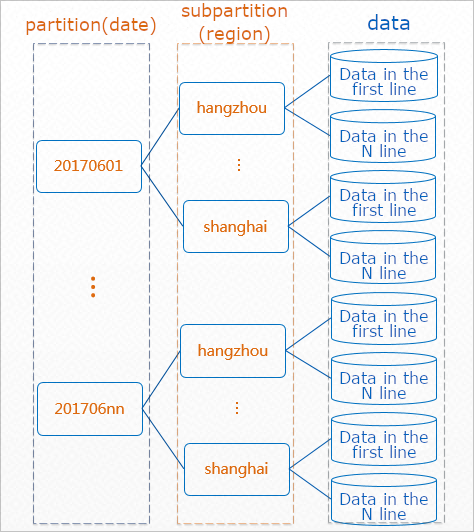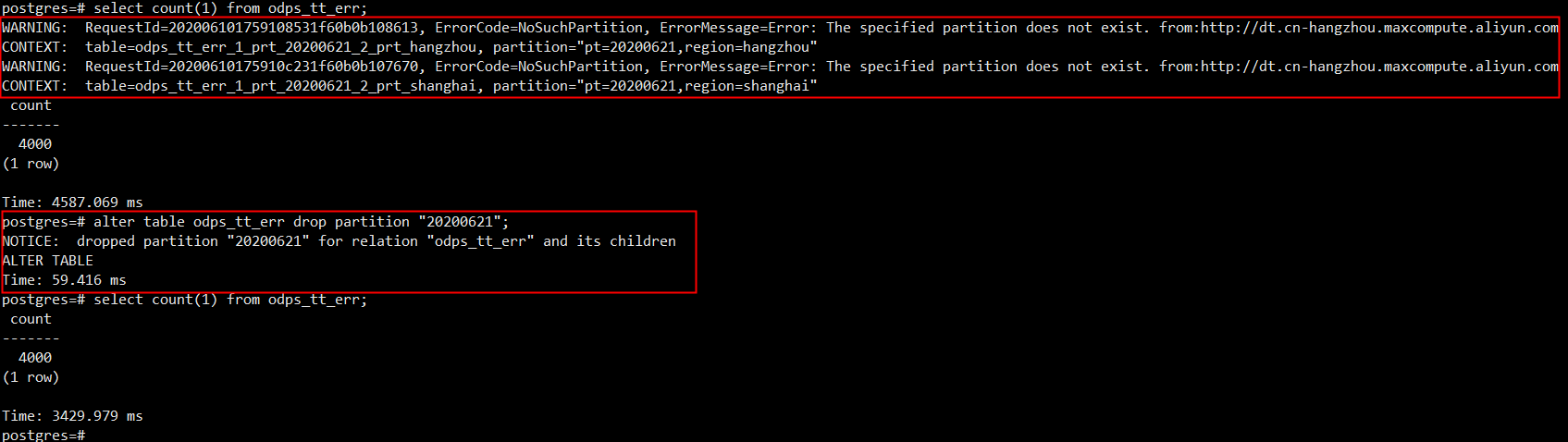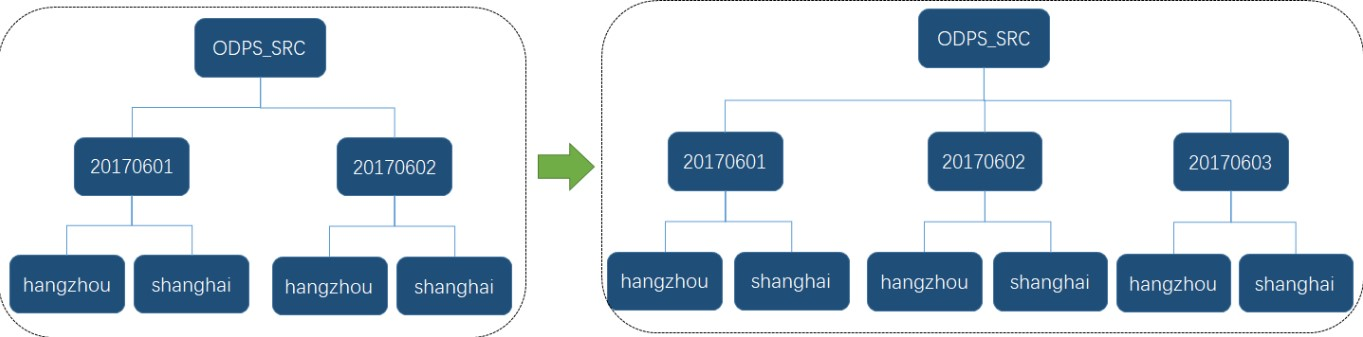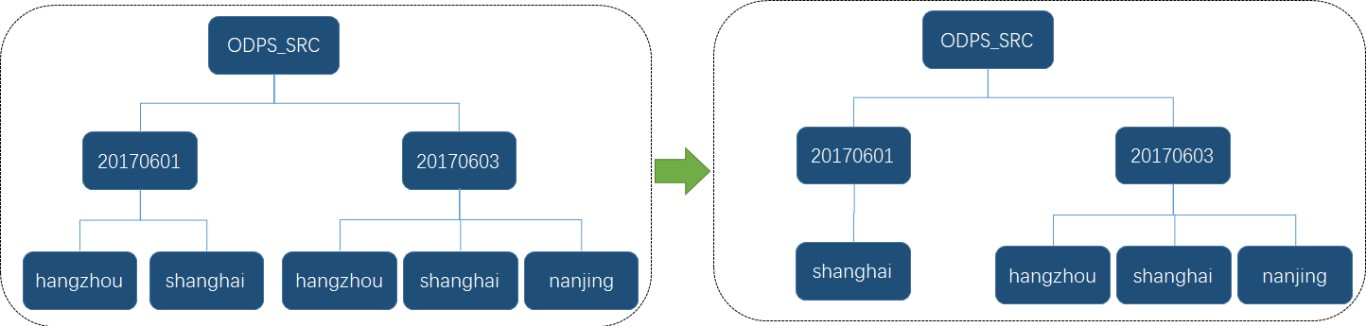Search
Document Center

# Use MaxCompute foreign tables to access MaxCompute data

Last Updated: Nov 02, 2021

MaxCompute foreign-data wrapper (FDW) of AnalyticDB for PostgreSQL is designed based on the PostgreSQL FDW framework to access data that is stored in MaxCompute.

MaxCompute FDW allows you to synchronize data from MaxCompute to AnalyticDB for PostgreSQL. You can create the following three types of MaxCompute foreign tables by using MaxCompute FDW:

• Non-partitioned foreign tables, which are mapped to non-partitioned MaxCompute tables.

• Last-level partition foreign tables, which are mapped to the last-level partitions of partitioned MaxCompute tables.

• Partitioned foreign tables, which are mapped to partitioned MaxCompute tables.

## Use MaxCompute FDW

1. Create the MaxCompute FDW extension in an AnalyticDB for PostgreSQL database.

``CREATE EXTENSION odps_fdw ;``

2. Grant the permission of using MaxCompute FDW to all database accounts.

``GRANT USAGE ON FOREIGN DATA WRAPPER odps_fdw TO PUBLIC;``
1. For a new AnalyticDB for PostgreSQL instance, the MaxCompute FDW extension is automatically created. In this case, you can skip the preceding steps.

2. For an existing AnalyticDB for PostgreSQL instance, you can use the privileged database account to connect to a specific database, and run the preceding commands to create the MaxCompute FDW extension and grant all database accounts the permission to use the extension.

## Use a MaxCompute foreign table

Before you use a MaxCompute foreign table, you must perform the following operations:

• Create a MaxCompute server to specify the endpoint for accessing MaxCompute.

• Create a MaxCompute user mapping to specify the account that can access the created MaxCompute server.

• Create a MaxCompute foreign table to specify the MaxCompute table that you want to access.

## 1. 1. Create a MaxCompute server

1.1 Sample command

``````CREATE SERVER odps_serv                        -- The name of the MaxCompute server
FOREIGN DATA WRAPPER odps_fdw
OPTIONS (
tunnel_endpoint '<odps tunnel endpoint>'   -- The endpoint of the MaxCompute Tunnel service
);``````

1.2 Options

You need to specify only one of the tunnel_endpoint and odps_endpoint options when you create a MaxCompute server in AnalyticDB for PostgreSQL. The following table describes these options.

Option

Required

Description

tunnel_endpoint

No. We recommend that you specify this option.

The endpoint of the MaxCompute Tunnel service.

odps_endpoint

No.

The endpoint of the MaxCompute service.

Note
• When you create a MaxCompute server, you can specify one or both of these options. If both options are specified, the specified MaxCompute Tunnel endpoint takes precedence. If the MaxCompute Tunnel endpoint is not specified, the MaxCompute endpoint is used to route access requests to the corresponding MaxCompute Tunnel endpoint.

• We recommend that you use the MaxCompute Tunnel endpoint in the Alibaba Cloud classic network or a VPC.

• When you use the Tunnel endpoint to access data over the Internet, you are charged USD 0.1166 for each GB of data.

## 2. 2. Create a user mapping to a MaxCompute server

2.1 Sample command

``````CREATE USER MAPPING FOR { username | USER | CURRENT_USER | PUBLIC }
SERVER odps_serv                                  -- The name of the MaxCompute server
OPTIONS (
id '<odps access id>',                                -- The AccessKey ID of the account used to access the MaxCompute server
key '<odps access key>'                               -- The AccessKey secret of the account used to access the MaxCompute server
);``````
Note

• username specifies the name of an existing account to map to the MaxCompute server.

• CURRENT_USER or USER specifies the name of the current user.

• If PUBLIC is specified, a public mapping is created. When no user-specific mapping is applicable, the public mapping is used.

2.2 Options

You must specify the type, the AccessKey ID, and the AccessKey secret of an AnalyticDB for PostgreSQL account that is used to access the MaxCompute server.

Option

Required

Description

`id`

Yes

The AccessKey ID of the account.

`key`

Yes

The AccessKey secret of the account.

## 3. 3. Create a MaxCompute foreign table

3.1 Sample command

``````CREATE FOREIGN TABLE IF NOT EXISTS table_name ( -- The name of the MaxCompute foreign table
column_name data_type [, ... ]
)
SERVER odps_serv                              -- The name of the MaxCompute server
OPTIONS (
project '<odps project>',                   -- The name of the MaxCompute project
table '<odps table>'                        -- The name of the MaxCompute table
);``````

3.2 Options

You can create a MaxCompute foreign table after you create a MaxCompute server and a user mapping to the MaxCompute server. The following table describes the options.

Option

Required

Description

`project`

Yes

The MaxCompute project. A project is a basic unit of MaxCompute. Similar to a database or schema in a traditional database system, a project is used to isolate users and control access requests. For more information, see Project.

`table`

Yes

The MaxCompute table. MaxCompute stores data in tables. For more information, see Table.

`partition`

No

The last-level partition in the partitioned MaxCompute table. Partitioning refers to dividing data in a table into independent parts based on partition keys. A partition key can be a single column or a combination of multiple columns. If a table is not partitioned, data is stored in the directory that stores the table. If a table is partitioned, each partition corresponds to a subdirectory in the directory that stores the table. In this case, data is stored in separate subdirectories. For more information about partitions, see Partition.

3.3 Types of foreign tables

MaxCompute FDW allows you to create the following three types of MaxCompute foreign tables based on the types of MaxCompute tables.

• Non-partitioned foreign tables

A non-partitioned foreign table is mapped to a non-partitioned MaxCompute table. When you create a non-partitioned foreign table, you need only to specify valid values for the project and table options. You do not need to specify the partition option, or you can leave the partition option empty. Example:

``````CREATE FOREIGN TABLE odps_lineitem (              -- The name of the MaxCompute foreign table
l_orderkey      bigint,
l_partkey       bigint,
l_suppkey       bigint,
l_linenumber    bigint,
l_quantity      double precision,
l_extendedprice double precision,
l_discount      double precision,
l_tax           double precision,
l_returnflag    char(1),
l_linestatus    char(1),
l_shipdate      date,
l_commitdate    date,
l_receiptdate   date,
l_shipinstruct  char(25),
l_shipmode      char(10),
l_comment       varchar(44)
) SERVER odps_serv                              -- The name of the MaxCompute server
OPTIONS (
project 'odps_fdw',                           -- The name of the MaxCompute project
table 'lineitem_big'                          -- The name of the MaxCompute table
);``````
• Last-level partition foreign tables

A last-level partition foreign table is mapped to a last-level partition of a MaxCompute table. When you create a last-level partition foreign table, you must specify valid values for the partition option. If a MaxCompute table is partitioned in multiple levels, you can map the last-level partition foreign table only to a last-level partition of the MaxCompute table. You must set the partition option to the full path of the last-level partition.

In the following example, a partitioned table that contains two levels of partitions in MaxCompute is created:

``````-- Create a partitioned table that contains two levels of partitions. The partition is based on the date and the subpartition is based on the region.
CREATE TABLE src (key string, value bigint) PARTITIONED BY (pt string,region string);``````For example, assume that a MaxCompute table is partitioned in two levels and contains the 20170601 partition and the Hangzhou subpartition. To create a last-level partition foreign table that is mapped to the Hangzhou subpartition in an AnalyticDB for PostgreSQL database, the partition option must be set to pt=20170601,region=hangzhou.

``````CREATE FOREIGN TABLE odps_src_20170601_hangzhou (   -- The name of the MaxCompute foreign table
key string,
value bigint
) SERVER odps_serv                                  -- The name of the MaxCompute server
OPTIONS (
project 'odps_fdw',                               -- The name of the MaxCompute project
table 'src',                                      -- The name of the MaxCompute table
partition 'pt=20170601,region=hangzhou'           -- The full path of the last-level partition
);``````
Note

1. Set the partition option in the key=value format. To specify a last-level partition in a multi-level partitioned table, separate the key-value pairs with commas (,). Do not include spaces in the partition option.

2. You cannot map a last-level partition foreign table to a non-last-level partition of a MaxCompute table. In this example, you cannot set the partition option to `pt=20170601`, which indicates the path of the partition.

3. Make sure that you set the partition option to the full path of a last-level partition. In this example, you cannot set the `partition` option to region=Hangzhou, which indicates the relative path of the last-level partition.

• Partitioned foreign tables

A partitioned foreign table is mapped to a partitioned MaxCompute table. The following code shows how to use the preceding MaxCompute table src that contains two levels of partitions to create a partitioned foreign table. For more information, see Define table partitioning.

``````CREATE FOREIGN TABLE odps_src(                    -- The name of the MaxCompute foreign table
key text,
value bigint,
pt text,                                        -- The partition key of the MaxCompute foreign table
region text                                     -- The subpartition key of the MaxCompute foreign table
) SERVER odps_serv
OPTIONS (
project 'odps_fdw',                             -- The name of the MaxCompute project
table 'src'                                     -- The name of the partitioned MaxCompute table
)
PARTITION BY LIST (pt)                            -- Use the pt column as the partition key
SUBPARTITION BY LIST (region)                     -- Use the region column as the subpartition key
SUBPARTITION TEMPLATE (                       -- The template of the subpartition
SUBPARTITION hangzhou VALUES ('hangzhou'),
SUBPARTITION shanghai VALUES ('shanghai')
)
( PARTITION "20170601" VALUES ('20170601'),
PARTITION "20170602" VALUES ('20170602'));``````
Note

When you create a partitioned foreign table in an AnalyticDB for PostgreSQL database, make sure that the following requirements are met. These requirements are different from those on the creation of partitioned tables in MaxCompute.

1. Append the partition keys as fields to the end of other fields. If you create a multi-level partitioned foreign table, the partition key order, the level of partition keys, and the partition levels of the MaxCompute table must match one another.

2. When you create a partitioned table, you must specify the partition key values. Use the LIST method to partition a table.

3. When you create a partitioned foreign table, you do not need to specify the partition option. If the partition option is specified, the foreign table is created based on the specified last-level partition of the specified MaxCompute table instead of the entire MaxCompute table.

4. If a partitioned foreign table contains partitions or subpartitions that have no matches in the specified MaxCompute table, an alert is triggered when you query the foreign table. In this case, you can delete the corresponding partition or subpartition from the foreign table. For more information, see the "Delete partitions or subpartitions from a partitioned foreign table" section in this topic.3.4 Add partitions or subpartitions to a partitioned foreign table

In this example, the preceding partitioned foreign table odps_src is used.

• Add a partition, as shown in the following figure.

``````-- Add a partition. The subpartitions are automatically created.
alter table odps_src add partition "20170603" values(20170603);``````• Add a subpartition, as shown in the following figure.

``````-- Add a subpartition.
alter table odps_src alter partition "20170603" add partition "nanjing" values('nanjing');``````3.5 Delete partitions or subpartitions from a partitioned foreign table

In this example, the preceding partitioned foreign table odps_src is used.

• Delete a partition, as shown in the following figure.

``````-- Delete a partition. The cascaded subpartitions are also deleted.
alter table odps_src drop partition "20170602";``````• Delete a subpartition, as shown in the following figure.

``````-- Delete a subpartition.
alter table odps_src alter partition "20170601" drop partition "hangzhou";``````## Data types supported by MaxCompute foreign tables

The following table lists the data type mappings between MaxCompute and AnalyticDB for PostgreSQL. We recommend that you specify the data types of columns in a foreign table in an AnalyticDB for PostgreSQL database based on this table.

Note

AnalyticDB for PostgreSQL does not support data types that correspond to the STRUCT, MAP, and ARRAY data types that are supported by MaxCompute.

MaxCompute data type

AnalyticDB for PostgreSQL data type

BOOLEAN

bool

TINYINT

int2

SMALLINT

int2

INTEGER

int4

BIGINT

int8

FLOAT

float4

DOUBLE

float8

DECIMAL

numeric

BINARY

bytea

VARCHAR(n)

varchar(n)

CHAR(n)

char(n)

STRING

text

DATE

date

DATETIME

timestamp

TIMESTAMP

timestamp

## Scenarios

You can execute foreign scans on AnalyticDB for PostgreSQL databases to scan MaxCompute foreign tables. Therefore, you can use the same query statements to query data in foreign tables and data in other tables in AnalyticDB for PostgreSQL. In this example, TPC Benchmark™H (TPC-H) queries are used to illustrate common scenarios where MaxCompute foreign tables are used.

## Query a MaxCompute foreign table

TPC-H Q1 queries are used to aggregate and filter data in a single table. In this example, a Q1 query is performed on the MaxCompute foreign table odps_lineitem.

``````-- Create the MaxCompute foreign table odps_lineitem.
CREATE FOREIGN TABLE odps_lineitem (
l_orderkey bigint,
l_partkey bigint,
l_suppkey bigint,
l_linenumber bigint,
l_quantity double precision,
l_extendedprice double precision,
l_discount double precision,
l_tax double precision,
l_returnflag CHAR(1),
l_linestatus CHAR(1),
l_shipdate DATE,
l_commitdate DATE,
l_receiptdate DATE,
l_shipinstruct CHAR(25),
l_shipmode CHAR(10),
l_comment VARCHAR(44)
) server odps_serv
options (
project 'odps_fdw', table 'lineitem'
);

-- TPC-H Q1
select
l_returnflag,
l_linestatus,
sum(l_quantity) as sum_qty,
sum(l_extendedprice) as sum_base_price,
sum(l_extendedprice * (1 - l_discount)) as sum_disc_price,
sum(l_extendedprice * (1 - l_discount) * (1 + l_tax)) as sum_charge,
avg(l_quantity) as avg_qty,
avg(l_extendedprice) as avg_price,
avg(l_discount) as avg_disc,
count(*) as count_order
from
odps_lineitem
where
l_shipdate <= date '1998-12-01' - interval '88' day --(3)
group by
l_returnflag,
l_linestatus
order by
l_returnflag,
l_linestatus;``````

## Import MaxCompute data to an on-premises table

Perform the following steps to import data:

1. Create a MaxCompute foreign table in an AnalyticDB for PostgreSQL database.

2. Execute one of the following statements to import data to the new table:

``````-- INSERT statement
INSERT INTO <Destination on-premises table> SELECT * FROM <MaxCompute foreign table>;

-- CREATE TABLE AS statement
CREATE TABLE <Destination on-premises table> AS SELECT * FROM <MaxCompute foreign table>;``````
• Example 1: Execute the INSERT statement to import the data in odps_lineitem to an on-premises append-optimized column-oriented storage (AOCS) table.

``````-- Create an on-premises AOCS table.
CREATE TABLE aocs_lineitem (
l_orderkey bigint,
l_partkey bigint,
l_suppkey bigint,
l_linenumber bigint,
l_quantity double precision,
l_extendedprice double precision,
l_discount double precision,
l_tax double precision,
l_returnflag CHAR(1),
l_linestatus CHAR(1),
l_shipdate DATE,
l_commitdate DATE,
l_receiptdate DATE,
l_shipinstruct CHAR(25),
l_shipmode CHAR(10),
l_comment VARCHAR(44)
) WITH (APPENDONLY=TRUE, ORIENTATION=COLUMN, COMPRESSTYPE=ZSTD, COMPRESSLEVEL=5)
DISTRIBUTED BY (l_orderkey);

-- Import the data in odps_lineitem_orc to the on-premises AOCS table.
INSERT INTO aocs_lineitem SELECT * FROM odps_lineitem;``````
• Example 2: Execute the CREATE TABLE AS statement to import the data in odps_lineitem to an on-premises heap table.

``create table heap_lineitem as select * from odps_lineitem distributed by (l_orderkey);``

## Associate a MaxCompute foreign table with an on-premises table

In this example, a TPC-H Q19 query is performed on the MaxCompute foreign table odps_part that is associated with the on-premises AOCS table aocs_lineitem.

``````-- TPC-H Q19
select
sum(l_extendedprice* (1 - l_discount)) as revenue
from
aocs_lineitem,          -- The name of the on-premises AOCS table
odps_part               -- The name of the MaxCompute foreign table
where
(
p_partkey = l_partkey
and p_brand = 'Brand#32'
and p_container in ('SM CASE', 'SM BOX', 'SM PACK', 'SM PKG')
and l_quantity >= 8 and l_quantity <= 8 + 10
and p_size between 1 and 5
and l_shipmode in ('AIR', 'AIR REG')
and l_shipinstruct = 'DELIVER IN PERSON'
)
or
(
p_partkey = l_partkey
and p_brand = 'Brand#41'
and p_container in ('MED BAG', 'MED BOX', 'MED PKG', 'MED PACK')
and l_quantity >= 15 and l_quantity <= 15 + 10
and p_size between 1 and 10
and l_shipmode in ('AIR', 'AIR REG')
and l_shipinstruct = 'DELIVER IN PERSON'
)
or
(
p_partkey = l_partkey
and p_brand = 'Brand#44'
and p_container in ('LG CASE', 'LG BOX', 'LG PACK', 'LG PKG')
and l_quantity >= 22 and l_quantity <= 22 + 10
and p_size between 1 and 15
and l_shipmode in ('AIR', 'AIR REG')
and l_shipinstruct = 'DELIVER IN PERSON'
);``````

## Common errors in using MaxCompute foreign tables

Tunnel Common Errors

## Notes

You can synchronize data from MaxCompute to MaxCompute foreign tables by using MaxCompute Tunnel. The synchronization performance is subject to the server resources and the outbound network bandwidth of MaxCompute Tunnel. Therefore, we recommend that you take note of the following items:

1. If you use only foreign tables, an ApsaraDB for PostgreSQL database can contain a maximum of five foreign tables.

2. If you associate multiple MaxCompute foreign tables, import the data in large MaxCompute foreign tables to on-premises tables and then associate the on-premises tables with small foreign tables to improve the performance.